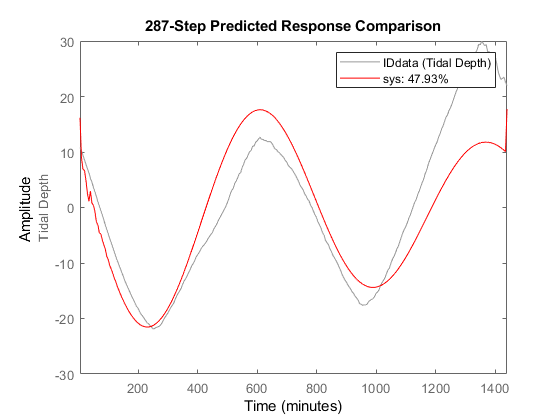## Visualize and Compare Measured and Predicted Tidal Depths

This example shows how to compare the measured and predicted tidal depth at Ockway Bay. Predicting tidal depths is vital: if you are not aware of the water depth, your boat can get easily stuck in the mud in a shallow bay.

### Read Data from the Ockway Bay Real-Time Tide Gauge

ThingSpeak™ channel 50289 contains data about tidal depth at Ockway Bay. The data is collected once every 5 minutes. Field 1 of the channel contains tidal depth data. Read the data using the `thingSpeakRead` function from channel 50289 on a particular day, for example, July 01, 2016.

```startDate = datetime('July 1, 2016 12:01:00 AM'); endDate = datetime('July 2, 2016 12:02:00 AM'); dateRange = startDate:endDate; data = thingSpeakRead(50289,'DateRange',dateRange,'Fields',1); ```

### Create Data Object and Detrend the Data

Use the `iddata` function to create an `iddata` object of the tidal-depth data. As the tidal data is not zero mean, use `detrend` to make the data have a zero mean.

NOTE: This example is designed to illustrate how an AR model can be used to model a sinusoidal function such as tide level. It is not designed as an alternative to sophisticated tidal forecasting techniques. MATLAB® functions that forecast tide levels using measured tidal data, such as UTide functions are available on MATLAB Central.

```sampleTime = 5; IDdata = iddata(data,[],sampleTime,'OutputName',{'Tidal Depth'},'TimeUnit','minutes') IDdata = detrend(IDdata,0); ```
```IDdata = Time domain data set with 288 samples. Sample time: 5 minutes Outputs Unit (if specified) Tidal Depth ```

### Fit an AR Model to the Data

Since the tidal depth varies with time, use the `ar` function to fit a discrete-time autoregressive model to the data.

```modelOrder = 8; sys = ar(IDdata,modelOrder); ```

### Compare the Measured and Predicted Tidal Depths

Use the `compare` function to compare the accuracy of the predicted model data to that of the measured data.

```compare(IDdata,sys,287,'r') ```This plot shows how the 287-step-ahead predicted response of the system compares to the measured data.